### FOCUS AREAS

Contents | SAS Program | PDF

# The %MktOrth Macro

## Introduction

The %MktOrth autocall macro lists some of the 100% orthogonal main-effects plans that the %MktEx macro can generate.

### %MktOrth Macro Syntax

%MktOrth( <optional arguments>)

#### Optional Arguments

FILTER=n

specifies extra design catalog filtering. Usually, you will never have to use this argument. By default, the %MktOrth macro filters out inferior designs. On the one hand, this process is expensive, but it also saves some time and resources by limiting the information that must be processed. Care is taken to do only the minimum amount of work to filter. However, this gets complicated. If you specify MAXLEV=144, the maximum, you get perfect filtering of duplicates in a reasonable amount of time. The %MktOrth macro uses internal and optimized constants to avoid doing extra work. Unfortunately, these constants might not be optimal for any other MAXLEV= argument value. This will almost never be a real issue. However, if you want to both specify the MAXLEV= argument and ensure that you get better filtering, then specify FILTER=(for some) and you might get a few more inferior designs filtered out. Thevalue increases how deeply into the catalog the %MktOrth macro searches for inferior designs. Larger values find more designs to exclude, but at a cost of greater run time. The following steps use both the default filtering and specify FILTER=1000:

```%mktorth(maxlev=20)
%mktorth(maxlev=20, filter=1000)
```

The latter specification removes on the order of 30 more designs, but at the cost of a much slower run time. Actually, FILTER=27 is large enough for this example, and it has a negligible effect on run time, but you have to run the macro multiple times to figure that out. Values of a couple hundred or so are probably always going to be sufficient.

MAXLEV=n

specifies the maximum number of levels to consider. Specify a value, such that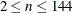. By default, MAXLEV=50. This argument controls the number of `x` variables in the OUTLEV= data set. It also excludes from consideration designs that have factors of more than n levels, so it affects the number of rows in the output data sets. Note that specifying MAXLEV=n does not preclude designs that have more than n-level factors from being used as parents for other designs; it just prevents the larger designs from being output. For example, when MAXLEV=3, the design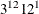in 36 runs is used to make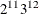before thedesign is discarded. Specifying smaller values makes the macro run faster.

MAXN=n

specifies the maximum number of runs of interest. Specifying small numbers (such as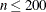) makes the macro run faster.

OPTIONS=options-list

specifies binary arguments. You can specify one or more of the following values:

LINEAGE

constructs the design lineage, which is the set of instructions about how the design is made.

MKTEX

specifies that the macro is being called from the %MktEx macro and only the OUTLEV= data set is needed. The macro takes shortcuts to make it run faster, doing only what the %MktEx macro needs.

MKTRUNS

specifies that the macro is being called from the %MktRuns macro and only the OUTLEV= data set is needed. The macro takes shortcuts to make it run faster, doing only what the %MktRuns macro needs.

PARENT

specifies that only parent designs should be listed.

DUPS

specifies that the %MktRuns macro should not filter out duplicate and inferior designs from the catalog. This can be useful when you are creating a data set for the CAT= argument in the %MktEx macro.

512

adds some larger designs in 512 runs with mixes of 16-, 8-, 4-, and 2-level factors to the catalog, giving added flexibility in 512 runs at a cost of much slower run time. This argument replaces the default parent design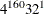with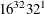.

By default, none of these arguments are specified.

OUTALL=SAS-data-set

specifies the output data set that contains all designs. This data set is not created by default. The data set is like the OUTLEV= data set, except larger. The OUTALL= data set includes all of the %MktEx macro design catalog, including all the smaller designs that can be trivially made from larger designs by dropping factors. For example, when the OUTLEV= data set has `X2`=2 `X3`=2, the OUTALL= data set has that design and also `X1`=2 `X3`=1, `X1`=1 `X3`=2, and `X1`=1 `X2`=1. When you specify OUTALL=, you must also specify a reasonably small value of the RANGE= or MAXN= argument. Otherwise, the OUTALL= specification will take a long time and create a huge data set, which will very likely be too large to store on your computer.

OUTCAT=SAS-data-set

specifies the output data set that contains the catalog of designs that the %MktEx macro can create. By default, OUTCAT=`MktDesCat`.

OUTLEV=SAS-data-set

specifies the output data set that contains the list of designs and 50 (by default) more variables, `X1–X50`, which includes `X2` (the number of 2-level factors), `X3` (the number of 3-level factors), and so on. By default, OUTLEV=`MktDesLev`. The number of `X` variables is determined by the MAXLEV= argument.

RANGE=range-specification

specifies the number of runs of interest. Specify a range that involves n, where n is the number of runs. Your range specification must be a logical expression that involves n. For example:

```RANGE=n=36
RANGE=18 le n le 36
RANGE=n eq 18 or n eq 36
```

### Help Option

You can specify either of the following to display the argument names and simple examples of the macro syntax:

```%mktorth(help)
%mktorth(?)
```

### %MktOrth Macro Notes

This macro specifies `options nonotes` throughout most of its execution. If you want to see all the notes, submit the following statement before running the macro:

```%let mktopts = notes;
```

To see the macro version, submit the following statement before running the macro:

```%let mktopts = version;
```

### Examples

The %MktOrth macro is usually used indirectly; it is called by the %MktEx macro. However, you can directly call the %MktOrth macro to see what orthogonal designs are available and decide which ones to use. The following statement requests all the designs in the catalog that have 100 or fewer runs and 2-level through 6-level factors (with no higher-level factors):

```%mktorth(maxn=100, maxlev=6)
```

The macro creates data sets and displays no output except the following notes:

```NOTE: The data set WORK.MKTDESLEV has 357 observations and 9 variables.
NOTE: The data set WORK.MKTDESCAT has 357 observations and 3 variables.
```

The next statement generates the entire catalog of 121,270 designs, including more than 62,000 designs in 512 runs that are not generated by default:

```%mktorth(maxlev=144, options=512)
```

The next statement generates the catalog of 58,690 designs, including designs that have up to 144-level factors:

```%mktorth(maxlev=144)
```

Unless you really want to see all the designs, you can make the %MktOrth macro run much faster by specifying smaller values for the RANGE= or MAXN= argument (to control the number of runs) and the MAXLEV= argument (to control the maximum number of factor levels and the number of variables in the MKTDESLEV data set) than the defaults (RANGE= N LE 1000, MAXN=1000, MAXLEV=50). The maximum number of levels that you can specify is 144.

The following statement lists the first few and last few designs in the catalog:

```proc print data=mktdeslev(where=(n le 12 or n ge 972));
var design reference;
id n;
by n;
run;
```

Some of the results are as follows:

```              n              Design              Reference

6    2 **  1  3 **  1              Full-Factorial

2 **  4            4 **  1    Fractional-Factorial

9             3 **  4              Fractional-Factorial

10    2 **  1            5 **  1    Full-Factorial

2 **  4  3 **  1              Orthogonal Array
2 **  2            6 **  1    Orthogonal Array
3 **  1   4 **  1    Full-Factorial

2 **972            4 **  1    Orthogonal Array
2 **969            4 **  2    Orthogonal Array
2 **968            8 **  1    Orthogonal Array
2 **966            4 **  3    Orthogonal Array

2 **980            4 **  1    Orthogonal Array

2 **988            4 **  1    Orthogonal Array
2 **985            4 **  2    Orthogonal Array
2 **984            8 **  1    Orthogonal Array
2 **982            4 **  3    Orthogonal Array
2 **979            4 **  4    Orthogonal Array
2 **976            4 **  5    Orthogonal Array
2 **976           16 **  1    Orthogonal Array
2 **973            4 **  6    Orthogonal Array
2 **970            4 **  7    Orthogonal Array

2 **996            4 **  1    Orthogonal Array
```

In most ways, the catalog stops at 513 runs. The exceptions include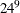in 576 runs,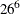in 676 runs, Hadamard designs up to= 1,000, and designs easily constructed from those Hadamard designs (by creating 4-level factors, 8-level factors, 16-level factors, and so on).

The following statement displays the first few designs and variables in the `MktDesLev` data set:

```proc print data=mktdeslev(where=(n le 12));
var design reference x1-x6;
id n;
by n;
run;
```

Some of the results are as follows:

```   n            Design            Reference             x1  x2  x3  x4  x5  x6

4  2 **  3                     Hadamard               0   3   0   0   0   0

6  2 **  1  3 **  1            Full-Factorial         0   1   1   0   0   0

8  2 **  7                     Hadamard               0   7   0   0   0   0
2 **  4            4 **  1  Fractional-Factorial   0   4   0   1   0   0

9           3 **  4            Fractional-Factorial   0   0   4   0   0   0

10  2 **  1            5 **  1  Full-Factorial         0   1   0   0   1   0

12  2 ** 11                     Hadamard               0  11   0   0   0   0
2 **  4  3 **  1            Orthogonal Array       0   4   1   0   0   0
2 **  2            6 **  1  Orthogonal Array       0   2   0   0   0   1
3 **  1   4 **  1  Full-Factorial         0   0   1   1   0   0

```

If you want to display only a list of designs, possibly selecting on the number of runs, you can use the `MktDesCat` data set. However, if you want to do more advanced processing, based on the numbers of levels of some of the factors, you can use the OUTLEV=`MktDesLev` data set to select potential designs. You can look at the level information in `MktDesLev` and see the number of 2-level factors in `X2`, the number of 3-level factors in `X3`, and so on, up to the number of 144-level factors in `X144`. The number of 1-level factors, `X1`, is always zero, but `X1` is available so you can make arrays (for example, array `x`) and have `x` refer to `X2`, the number of 2-level factors, and so on.

Suppose you are interested in the design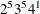. The following statements display some of the ways in which it is available:

```%mktorth(maxn=100)

proc print data=mktdeslev noobs;
where x2 ge 5 and x3 ge 5 and x4 ge 1;
var n design reference;
run;
```

Some of the results are as follows:

```          n                   Design                      Reference

72    2 ** 44  3 ** 12   4 **  1              Orthogonal Array
72    2 ** 43  3 **  8   4 **  1   6 **  1    Orthogonal Array
72    2 ** 37  3 ** 13   4 **  1              Orthogonal Array
72    2 ** 36  3 **  9   4 **  1   6 **  1    Orthogonal Array
72    2 ** 35  3 ** 12   4 **  1   6 **  1    Orthogonal Array
.
.
.

```

The following statements illustrate one way that you can see all the designs in a certain range of sizes:

```%mktorth(range=12 le n le 20)

proc print;
id n;
by n;
run;
```

The results are as follows:

```        n                   Design                   Reference

2 **  4  3 **  1                        Orthogonal Array
2 **  2            6 **  1              Orthogonal Array
3 **  1   4 **  1              Full-Factorial

14    2 **  1            7 **  1              Full-Factorial

15             3 **  1   5 **  1              Full-Factorial

2 ** 12            4 **  1              Fractional-Factorial
2 **  9            4 **  2              Fractional-Factorial
2 **  8            8 **  1              Fractional-Factorial
2 **  6            4 **  3              Fractional-Factorial
2 **  3            4 **  4              Fractional-Factorial
4 **  5              Fractional-Factorial

18    2 **  1  3 **  7                        Orthogonal Array
2 **  1            9 **  1              Full-Factorial
3 **  6   6 **  1              Orthogonal Array

2 **  8            5 **  1              Orthogonal Array
2 **  2           10 **  1              Orthogonal Array
4 **  1   5 **  1    Full-Factorial
```

The %MktOrth macro can output the lineage of each design, which is the set of steps that the %MktEx macro uses to create it. The following statements illustrate this option:

```%mktorth(range=n=36, options=lineage)

proc print noobs;
where index(design, '2 ** 11') and index(design, '3 ** 12');
run;
```

The results are as follows:

```             n         Design            Reference

36    2 ** 11  3 ** 12    Orthogonal Array

Lineage

36 ** 1 : 36 ** 1 > 3 ** 12 12 ** 1 : 12 ** 1 > 2 ** 11

```

The designin 36 runs starts out as a single 36-level factor,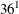. Thenis replaced by. Finally,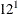is replaced by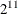, resulting in.